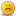# Thread: How to do populate values in Quality Center from values in Excel?

1. ## How to do populate values in Quality Center from values in Excel?

How to do populate values in Quality Center from values in Excel?

I am trying to populate a field in Quality Center from a field in an Excel spreadsheet based off what is entered in another field in Quality Center. My code doesnt populate anything and doesnt give me any errors. Can someone please help me?

Here is the code.

The code is
Sub Defects_Bug_FieldChange(FieldName)
Dim objExcel
Dim excelPath
Dim cells
Dim row
Dim sheet
Dim word
Dim objWorkbook

excelPath = "C:\Test\Quality Center\QATOwnersTest.xls" objExcel.Workbooks.open excelPath, false, true
Set objExcel = CreateObject("Excel.Application")
Set Sheet = objExcel.ActiveWorkBook.Worksheet(1)

IF (Bug_Fields("BG_USER_06").Value = "ABC" AND Bug_Fields("BG_USER_04").Value = "EFG") Then

For row = 1 To 3
IF Bug_Fields ("BG_USER_11").Value = Sheet.Cells(Row, 1) Then
Bug_Fields ("BG_USER_12").Value = Sheet.Cells(Row, 2)End IF

Next
End If
objExcel.QuitReply With Quote

2. ## Re: How to do populate values in Quality Center from values in Excel?

Hi snabors,

Based on your attached code, the code seems to be in place for checking whether BG_USER_11 field value equals the retrieved value from Row, col of excel and then populating BG_USER_12 with excel value.

I would suggest try debugging your code in the following manner. Also I see that "objExcel.Workbooks.open excelPath, false, true " line to be included prior to creating Excel Object. Is that so ?

excelPath = "C:\Test\Quality Center\QATOwnersTest.xls"
Set objExcel = CreateObject("Excel.Application")
objExcel.Workbooks.open excelPath, false, true
Set Sheet = objExcel.ActiveWorkBook.Worksheet(1)

IF (Bug_Fields("BG_USER_06").Value = "ABC" AND Bug_Fields("BG_USER_04").Value = "EFG") Then

msgbox "Values Matched"
For row = 1 To 3

set OutputValue = Sheet.Cells(Row, 1)
msgbox OutputValue

If Bug_Fields ("BG_USER_11").Value = OutputValue then
Bug_Fields ("BG_USER_12").Value = Sheet.Cells(Row, 2)
Msgbox "BG_USER_12 value set".
end if

next

End if

Also try to check whether the code actually enters the If conditions or not. Incase the condition never returns true then I guess your code will never do anything. Try including msgbox when you enter If Loop to reflect whether code has been reached or not.

Cheers....Reply With Quote

####Posting Permissions

• You may not post new threads
• You may not post replies
• You may not post attachments
• You may not edit your posts
•